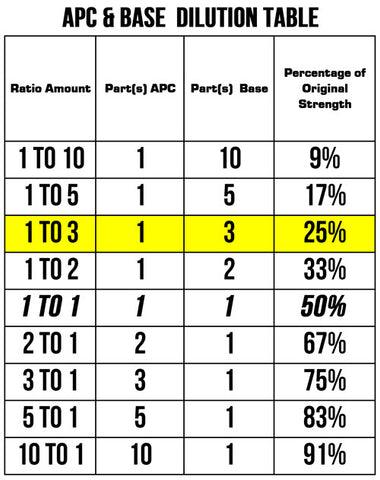# AP-Base All Purpose Base Cream 5 OZ Pump

\$19.99

AP-Base (All Purpose Base) is used to dilute the strength of other All Purpose Creams.

As an example:

If one wanted an APC to be 25% of its original strength, one could look at the chart below on the column "Percentage of Original Strength" and look down to the row with 25%.

This 25% row lists 1 Part APC and 3 Parts Base. This means whatever amount (teaspoon, pea size, dime size) of APC that is used, you want to use 3 times that amount (teaspoon, pea size, dime size) of the Base.If you know the desired strength, you can use the formula below to calculate the ratio of APC/Base.

Base Per APC = (APC%/ Desired %) - 1

Example:

If you are using APBP All Purpose Benzoyl Peroxide and want a 10% strength cream instead of the 25%, we can use the formula above to find out how much Base to use for each part of APBP.

APC% = 25% (Strength of APBP)

Desired% = 10% (The Strength We Want)

Using the formula above:

Base Per APC = (APC%/ Desired %) - 1

Base Per APC=  (.25/.10) - 1 = (2.5) - 1 = 1.5

So this means, if we have a 25% strength APC and want a 10% strength mixture, we would use 1.5 times the amount of base as whatever APC we had and mix them together.